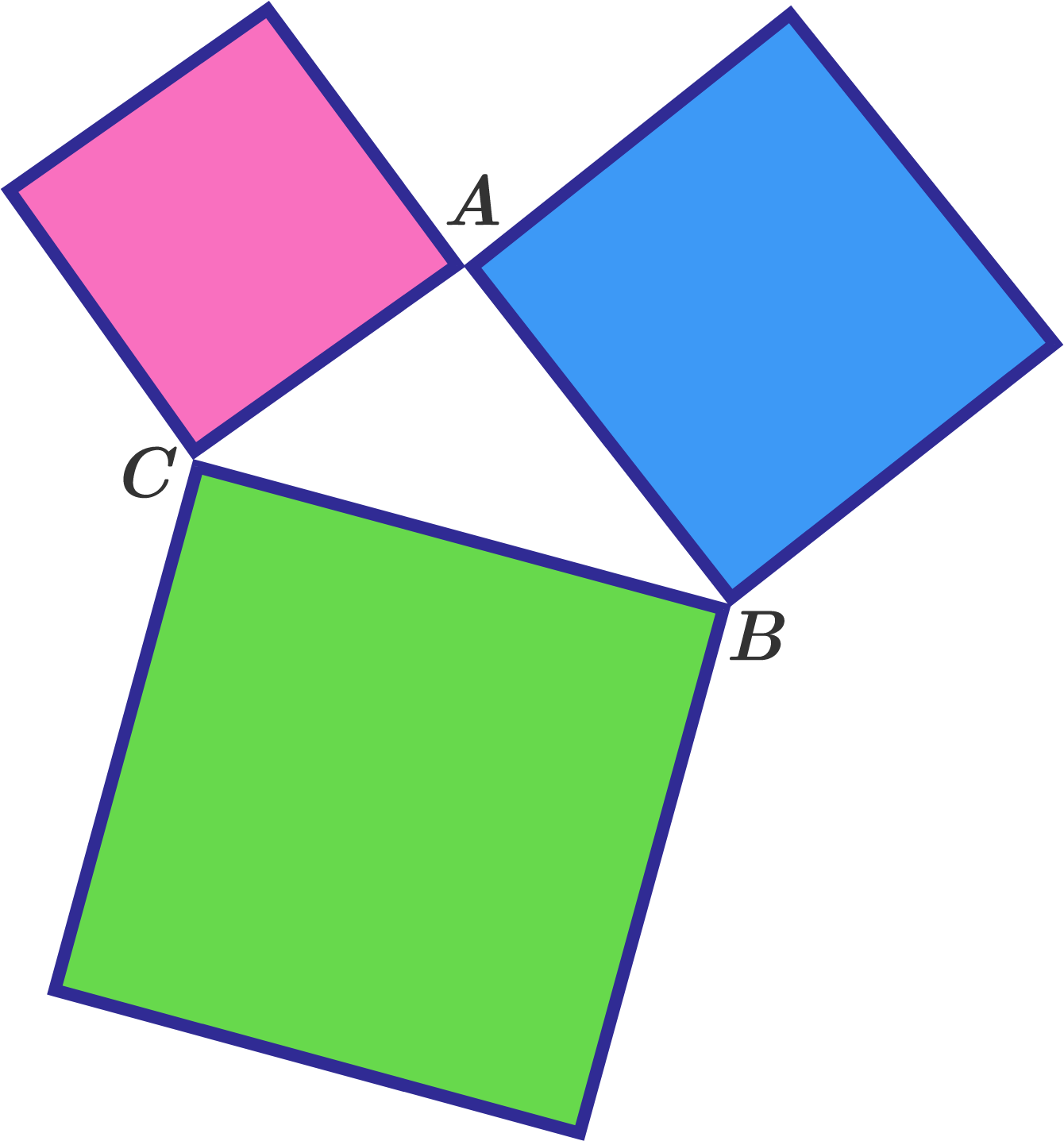# Three Squares Form A Triangle

Geometry Level 1Three squares are joined together as shown in figure to create a triangle.

What is the sum of angles $\angle A + \angle B + \angle C$ ?

Note: We are referring to the "exterior angles", not the interior angles of the triangle.

×